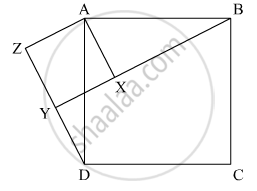# In the Given Figure, X is a Point in the Interior of Square Abcd. Axyz Is Also a Square. If Dy = 3 Cm And Az = 2 Cm, Then By = - Mathematics

MCQ

In the given figure, X is a point in the interior of square ABCD. AXYZ is also a square. If DY = 3 cm and AZ = 2 cm, then BY =• 5 cm

• 6 cm

• 7 cm

• 8 cm

#### Solution

In the following figure we are given

DY = 3cm

AZ = 2cm

Where ABCD is a square and AXYZ is also a square

We are asked to find BYFrom the above figure we have XY=YZ=AZ=AX

Now in the given figure

DZ = DY + YZ

= 3+2

= 5cm

So,

AD = sqrt(DZ^2 + AZ^2)

 = sqrt(25 + 4)

 = sqrt 29 cm

Now inn triangle ΔAXB

BX = sqrt(AB^2 - AX^2)

 = sqrt(29 - 4)

= 5cm

So

BY = XY + BX

= 2 + 5

=  7 cm

Concept: Congruence of Triangles
Is there an error in this question or solution?

#### APPEARS IN

RD Sharma Mathematics for Class 9
Chapter 12 Congruent Triangles
Exercise 12.8 | Q 19 | Page 87
Share